#Linear Inequalities in Two Variables

Need some basic understanding of sentences in two variables first? Introduction to Equations and Inequalities in Two Variables

Here's what this lesson offers:

## Going from Linear Equations to Linear Inequalities

You've already learned that the graph of $\,y = x + 1\,$ is the line shown below. This line is the picture of all the points $\,(x,y)\,$ that make the equation ‘$\,y=x+1\,$’ true.

How can ‘$\,y=x+1\,$’ be true? For a given $\,x$-value, the $\,y$-value must equal $\,x + 1\,.$ For each $\,x$-value, there is exactly one corresponding $\,y$-value—whatever $\,x\,$ is, plus $\,1\,.$

The line is the picture of all the points $\,(x,x+1)\,,$ as $\,x\,$ varies over all real numbers.

The line ‘$\,y=x+1\,$’ is all points of the form:

$$\bigl( \cssId{s16}{x\ \ ,} \cssId{s17}{\overbrace{x+1}^{\text{the y-value EQUALS x+1}}} \bigr)$$Graph of $\,y = x + 1\,$:
all points of the form $\,(x,x+1)$

Question: What happens if the verb in the sentence ‘$\,y \color{red}{=} x+1\,$’ is changed from ‘$\,\color{red}{=}\,$’ to $\,\lt\,,$  $\,\gt\,,$  $\,\le\,,$  or  $\,\ge\,$?

Answer: You go from a linear equation in two variables, to a linear inequality in two variables. The solution set changes dramatically! What was a line now becomes an entire half-plane: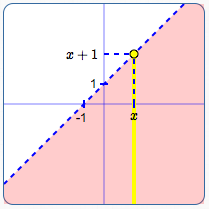Graph of $\,y < x + 1\,$:

All points of the form $\,(x,y)\,$ where the $y$-value is less than $\,x+1\,.$

Line is dashed; shade below the line.Graph of $\,y > x + 1\,$:

All points of the form $\,(x,y)\,$ where the $y$-value is greater than $\,x+1\,.$

Line is dashed; shade above the line.Graph of $\,y \le x + 1\,$:

All points of the form $\,(x,y)\,$ where the $y$-value is less than or equal to $\,x+1\,.$

Line is solid; also shade below the line.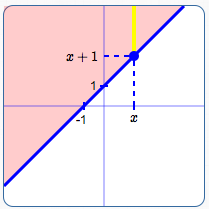Graph of $\,y \ge x + 1\,$:

All points of the form $\,(x,y)$ where the $y$-value is greater than or equal to $\,x+1\,.$

Line is solid; also shade above the line.

## Important Concepts for Graphing Linear Inequalities in Two Variables

### Definition: Linear Inequality in Two Variables

A linear inequality in two variables is a sentence of the form $$\cssId{s41}{ax + by + c < 0}\,,$$ where $\,a\,$ and $\,b\,$ are not both zero; $\,c\,$ can be any real number.

The inequality symbol can be any of these: $$\cssId{s45}{\lt\,,\ \ \gt\,,\ \ \le\,,\ \ \ge}$$

Remember:   ‘A sentence of the form ...’   really means   ‘A sentence that can be put in the form ...’

### Examples of Linear Inequalities in Two Variables:

$$\begin{gather} \cssId{s51}{3x - 4y + 5 \gt 0}\cr \cr \cssId{s52}{y \le 5x - 1}\cr \cr \cssId{s53}{x \ge 2}\cr \cssId{s54}{\text{(shorthand for \,x + 0y \ge 2\,)}}\cr \cr \cssId{s55}{y \lt 5}\cr \cssId{s56}{\text{(shorthand for \,0x + y \lt 5\,)}} \end{gather}$$

Key ideas for recognizing linear inequalities in two variables:

• The verb must be an inequality symbol: $$\cssId{s59}{\lt\,,\ \ \le\,,\ \ \gt\,,\ \ \text{ or } \ge}$$
• The variables must be raised only to the first power: no squares, no variables in denominators, no variables under square roots, and so on.
• You don't need to have both $\,x\,$ and $\,y\,,$ but you must have at least one of these variables.

### Linear Inequalities Graph as Half-Planes:

Every linear inequality in two variables graphs as a half-plane:

• If the verb is $\,\lt\,$ or $\,\gt\,,$ the boundary line is not included (dashed).
• If the verb is $\,\le\,$ or $\,\ge\,,$ the boundary line is included (solid).

If the linear inequality is in slope-intercept form (like $\,y \lt mx + b\,$), then it's easy to know which half of the line to shade:

• If the sentence is  $\,y \lt mx + b\,$  or  $\,y \le mx + b\,,$ shade below the line.
• If the sentence is  $\,y \gt mx + b\,$  or  $\,y \ge mx + b\,,$ shade above the line.

This only works if the inequality is in slope-intercept form! Of course, you can always put a sentence in slope-intercept form, by solving for $\,y\,.$ Then, you can use this method. But, the ‘test point method’ (below) is usually quicker-and-easier, if the sentence isn't already in slope-intercept form.

## The Test Point Method for Graphing Linear Inequalities in Two Variables

So, what about graphing something like $\,2x - y \lt 3\,,$ which isn't in slope-intercept form? You can (if desired) solve for $\,y\,,$ and then use the method above: $$\begin{gather} \cssId{sb3}{2x - y \lt 3}\cr \cssId{sb4}{-y \lt -2x + 3}\cr \cssId{sb5}{y \gt 2x - 3} \end{gather}$$ (Remember to change the direction of the inequality symbol when you multiply/divide by a negative number.)

The graph of $\,2x - y \lt 3\,$ is the same as the graph of $\,y \gt 2x - 3\,.$ Graph the line $\,y = 2x - 3\,$ (dashed), and then shade everything above (see below). However, there's an easier way. Keep reading!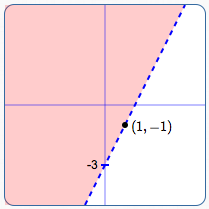Graph of $\,2x - y \lt 3\,$
(which is equivalent to $\,y > 2x - 3\,$)

The ‘Test Point Method’ is so-called because it involves choosing a ‘test point’ to decide which side of the line to shade. The process is illustrated with an example: graphing $\,2x - y \lt 3\,.$ The Test Point Method is usually easiest to use with sentences that aren't in slope-intercept form.

## Graph, Using the Test Point Method: $\,2x - y \lt 3$

### Step 1:  Identification

Recognize that ‘$\,2x - y \lt 3\,$’ is a linear inequality in two variables. Therefore, you know the graph is a half-plane. You need the boundary line; you need to know which side to shade.

### Step 2:  Boundary Line

Graph the boundary line $\,2x - y = 3\,$ using the intercept method:

• when $\,x = 0\,,$ $\,y = -3\,$
• when $\,y = 0\,,$ $\,x = \frac{3}{2}\,$

Since the verb in  ‘$\,2x - y \lt 3\,$’  is  ‘$\,<\,$’, this line is not included in the solution set. Therefore, the line is dashed.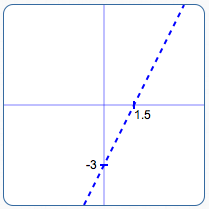Graph the boundary line
using the intercept method

### Step 3:  Test Point to Decide Which Side to Shade

Choose a simple point that is not on the line. Whenever $\,(0,0)\,$ is available, choose it! Zeroes are very easy to work with.

Is $\,(0,0)\,$ in the solution set? Substitute $\,x = 0\,$ and $\,y = 0\,$ into the original sentence ($\,2x - y \lt 3\,$), to see if it is true or false. Put a question mark over the inequality symbol, since you're asking a question:

$$\cssId{sb37}{2(0) - 0 \overset{?}{\lt} 3}$$

If the result is true, shade the side containing the test point. If the result is false, shade the other side.

Since ‘$\,0 < 3\,$’ is true, shade the side containing $\,(0,0)\,.$ Done!

With so many zeroes involved in this method, computations can often be done in your head, making this quick and easy!Choose test point $\,(0,0)\,$:
Since ‘$\,2(0) - 0 \lt 3\,$’ is true, shade the side containing the test point.

## Special Linear Inequalities in Two Variables: You only see one variable

Out of context, sentences like ‘$\,x \ge 2\,$’ and ‘$\,y \lt 5\,$’ can be confusing. You only see one variable, but that doesn't necessarily mean that there isn't another variable with a zero coefficient!

Out of context, here are clues to what is likely wanted:

• Solve: $\,x\ge 2\,$’ probably wants you to treat this as an inequality in one variable.
• Graph: $\,x\ge 2\,$’ probably wants you to treat this as an inequality in two variables.

As shown below, there is a big difference in the nature of the solution set!

Viewed as an inequality in one variable, the solution set of ‘$\,x\ge 2\,$’ is the set of all numbers that are greater than or equal to $\,2\,.$ The solution set is the interval $\,[2,\infty)\,,$ shown below.Graph of $\,x\ge 2\,,$
viewed as an inequality in one variable

Viewed as an inequality in two variables, ‘$\,x\ge 2\,$’ is really a shorthand for ‘$\,x + 0y \ge 2\,$’. The solution set is the set of all points $\,(x,y)\,,$ where the $x$-value is greater than or equal to $\,2\,.$ The $y$-value can be anything!

Here are examples of substitution into ‘$\,x + 0y \ge 2\,$’ :

• The point $\,(2,5)\,$ is in the solution set, since ‘$\,2 + 0(5) \ge 2\,$’ is true.
• The point $\,(3.5,-7.4)\,$ is in the solution set, since ‘$\,3.5 + 0(-7.4) \ge 2\,$’ is true.

The graph is the half-plane shown below. This is the picture of all the points with $x$-value greater than or equal to $\,2\,.$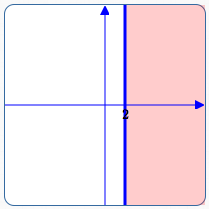Graph of $\,x\ge 2\,,$
viewed as an inequality in two variables

Viewed as an inequality in two variables, ‘$\,y\lt 5\,$’ is really a shorthand for ‘$\,0x + y \lt 5\,$’. The solution set is the set of all points $\,(x,y)\,,$ where the $y$-value is less than $\,5\,.$ The $x$-value can be anything!

Here are examples of substitution into ‘$\,0x + y \lt 5\,$’ :

• The point $\,(2,4)\,$ is in the solution set, since ‘$\,0(2) + 4 \lt 5\,$’ is true.
• The point $\,(-7.4,-3)\,$ is in the solution set, since ‘$\,0(-7.4) -3 \lt 5\,$’ is true.

The graph is the half-plane shown below. This is the picture of all the points with $y$-value less than $\,5\,.$Graph of $\,y\lt 5\,,$
viewed as an inequality in two variables